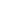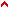1.1.1 Fig 1 The Master Figure
This part includes a figure called the master figure, and we call it the master figure because we will use it to investigate the truths of the other figures. It is composed of three squares and one circle, as shown.The minor square is divided into eight houses or cameras all equal in capacity, as shown, and it is contained in a circle, contained in turn by the major square signified by a. b. c. d. The circle stands in the middle between the major and minor squares and accordingly, it is signified by e. f. g. h. And the circle has the value of the square signified by i. k. l. m. n. o. p. q., plus the value of e. f. g. h.
In the middle between the biggest and smallest squares, stands a square signified by r. s. t. v. This is the square we are investigating as the one in which the circle is squared, inasmuch as the circle and the square are visibly equivalent in content and capacity; but we want to prove this with the art in the following way.
If the circle between the biggest and smallest squares stands equally between both, or in other words, as close to the one as to the other as does square r. s. t. v., then the circle must have 12 houses, and the square must have 12 equal houses as well. Given that the third square stands in the middle between the two squares as does the circle, the third square in the middle has the same value as the circle, and the circle is squared in it.
To further investigate the squaring of the circle, we now say that the number halfway between 8 and 16 units must have 12 units; and as the circle is in the middle between a square worth 16 units and a square worth 8 units, where all units are equal to each other, then the circle must be divided into 12 units which are equivalent to the sum of the units contained in the lesser square, plus its own units signified by e. f. g. h. Therefore, it is worth its own e. f. g. h. plus the value of i. k. l. m. n. o. p. q., whence it follows that the circle is worth the 4 lines, or sides enclosing i. k. l. m. n. o. p. q. inasmuch as these 4 lines are circumferential, and in addition, it is worth one of the four lines which make it worth more than the minor square, and this fifth line is the medium whereby the circle stands at an equal distance between a.b.c.d. and i.k.l.m.n.o.p.q.Top